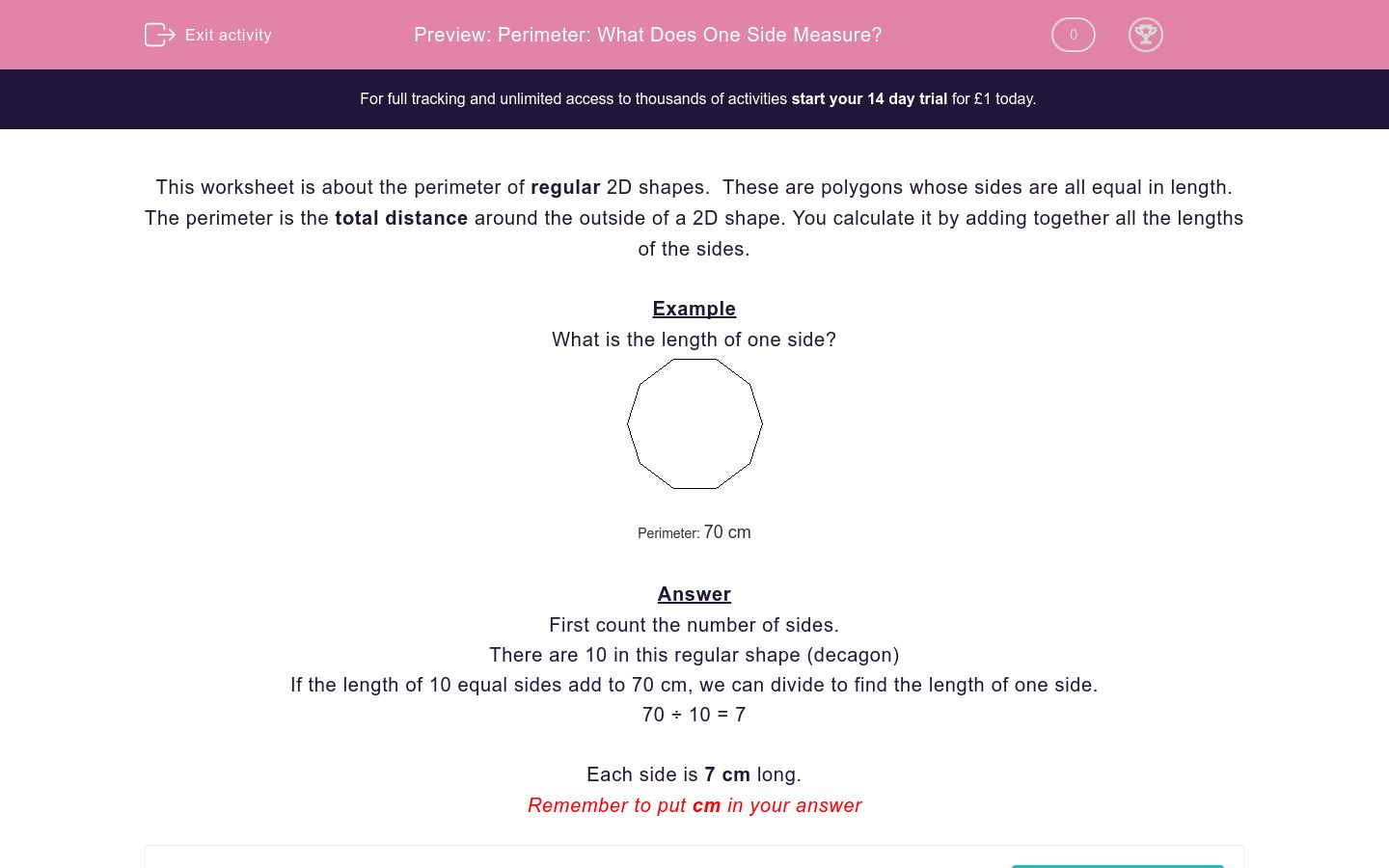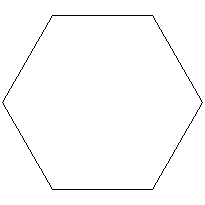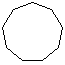# Perimeter: What Does One Side Measure?

In this worksheet, students calculate the length of one side of a regular polygon by first counting the number of sides and then dividing.Key stage:  KS 2

Curriculum topic:   Maths and Numerical Reasoning

Curriculum subtopic:   2D Shapes: Triangles, Quadrilaterals and Polygons

Difficulty level:### QUESTION 1 of 10

This worksheet is about the perimeter of regular 2D shapes.  These are polygons whose sides are all equal in length.

The perimeter is the total distance around the outside of a 2D shape. You calculate it by adding together all the lengths of the sides.

Example

What is the length of one side?Perimeter: 70 cm

First count the number of sides.

There are 10 in this regular shape (decagon)

If the length of 10 equal sides add to 70 cm, we can divide to find the length of one side.

70 ÷ 10 = 7

Each side is 7 cm long.

What is the length of one side?Perimeter: 20 cm

What is the length of one side?Perimeter: 60 cm

What is the length of one side?Perimeter: 80 cm

What is the length of one side?Perimeter: 27 cm

What is the length of one side?Perimeter: 48 cm

What is the length of one side?Perimeter: 90 cm

What is the length of one side?Perimeter: 60 cm

What is the length of one side?Perimeter: 63 cm

What is the length of one side?Perimeter: 72 cm
• Question 1

What is the length of one side?Perimeter: 20 cm
4cm
4 cm
• Question 2

What is the length of one side?Perimeter: 60 cm
10 cm
10cm
• Question 3

What is the length of one side?Perimeter: 80 cm
10cm
10 cm
• Question 4

What is the length of one side?Perimeter: 27 cm
3cm
3 cm
• Question 5

What is the length of one side?Perimeter: 48 cm
6 cm
6cm
• Question 6

What is the length of one side?Perimeter: 90 cm
10cm
10 cm
• Question 7

What is the length of one side?Perimeter: 60 cm
6 cm
6cm
• Question 8

What is the length of one side?Perimeter: 63 cm
9 cm
9cm
• Question 9

What is the length of one side?Perimeter: 72 cm
9cm
9 cm
---- OR ----

Sign up for a £1 trial so you can track and measure your child's progress on this activity.

### What is EdPlace?

We're your National Curriculum aligned online education content provider helping each child succeed in English, maths and science from year 1 to GCSE. With an EdPlace account you’ll be able to track and measure progress, helping each child achieve their best. We build confidence and attainment by personalising each child’s learning at a level that suits them.

Get started